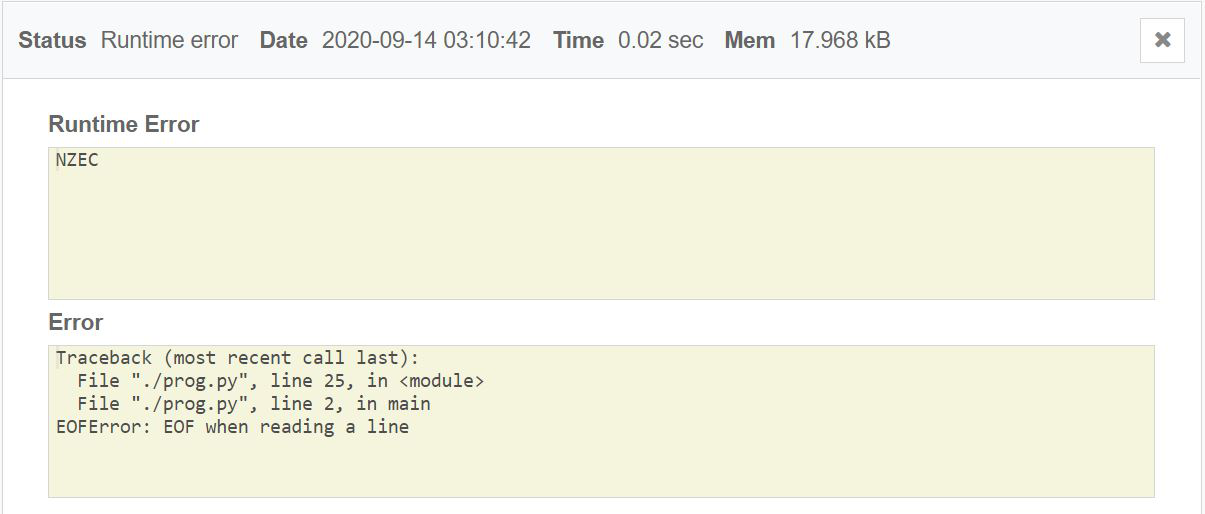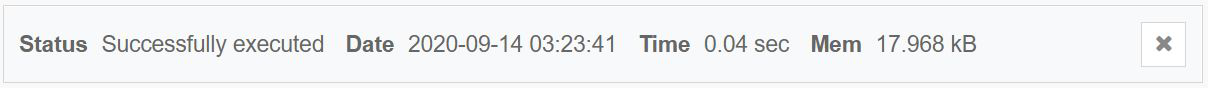Skip to content
Related Articles
Correcting EOF error in python in Codechef
• Last Updated : 24 Nov, 2020

EOF stands for End Of File. Well, technically it is not an error, rather an exception. This exception is raised when one of the built-in functions, most commonly input() returns End-Of-File (EOF) without reading any data.

EOF error is raised in Python in some specific scenarios:

• Sometimes all program tries to do is to fetch something and modify it. But when it is unable to fetch, it will raise this exception.
• When the input() function is interrupted in both Python 2.7 and Python 3.6+, or when the input() reaches the end of a file unexpectedly in Python 2.7.

All Built-in Exceptions in Python inherit from the BaseException class or extend from an inherited class therein. The full exception hierarchy of this error is:

BaseException -> Exception -> EOFError

The best practice to avoid EOF in python while coding on any platform is to catch the exception, and we don’t need to perform any action so, we just pass the exception using the keyword “pass” in the “except” block.

Consider the following code for the question in CodeChef K-Foldable String (KFOLD):

## C++

 `#Python program for the above question`` ` `#Function to reorder the characters``#of the string``def main():``    ``t = ``int``(input())``    ``while` `t:`` ` `        ``# Input variables ``        ``n, k = map(``int``, input().split())``        ``s = input()``        ``ans = ``""`` ` `        ``# Initialize dictionary``        ``s_dict = dict()``        ``for` `ch in s:``            ``s_dict[ch] = s_dict.get(ch, 0) + 1``        ``q = n``// k``        ``a1 = s_dict[``'1'``]``// q``        ``a0 = s_dict[``'0'``]``// q`` ` `        ``# Check for valid conditions``        ``if``(s_dict[``'1'``]%2!=0 or s_dict[``'0'``]%2!=0 \\``        ``or s_dict[``'1'``]%q!=0 or s_dict[``'0'``]%q!=0):``            ``ans = ``"Impossible"`` ` `        ``# Otherwise update the result``        ``else``:``            ``st = (``'0'``*a0) + (``'1'``*a1)``            ``st = (``'1'``*a1) + (``'0'``*a0)``            ``part1 = st + st_rev``            ``ans = part1*(q``// 2)`` ` `        ``# Print the result for the``        ``# current test case``        ``print(ans)`` ` `        ``t -= 1``    ``return`` ` `# Driver Code``if` `__name__==``"__main__"``:``    ``main()`

Output:

It gives the EOF error as shown below:The solution to the above EOF error is to enclose the code in try and except block and deal with exception accordingly, the approach to handle this exception is shown below:

## C++

 `# Python program for the above question`` ` `# Function to reorder the characters``#of the string``try` `: t = ``int``(input())`` ` `    ``# Input test cases``    ``while` `t:`` ` `        ``# Input Variables``        ``n, k = map(``int``, input().split())``        ``s = input()``        ``ans = ``""`` ` `        ``# Initialize dictionary``        ``s_dict = dict()``        ``for` `ch in s:``            ``s_dict[ch] = s_dict.get(ch, 0) + 1``        ``q = n``// k``        ``a1 = s_dict[``'1'``]``// q``        ``a0 = s_dict[``'0'``]``// q`` ` `        ``# Check for valid conditions``        ``if``(s_dict[``'1'``]%2!=0 or s_dict[``'0'``]%2!=0 \\ ``        ``or s_dict[``'1'``]%q!=0 or s_dict[``'0'``]%q!=0):``            ``ans = ``"Impossible"``  ` `        ``# Otherwise update the result``        ``else``:``            ``st = (``'0'``*a0) + (``'1'``*a1)``            ``st = (``'1'``*a1) + (``'0'``*a0)``            ``part1 = st + st_rev``            ``ans = part1*(q``// 2)`` ` `        ``# Print the result for the``        ``# current test case``        ``print(ans)`` ` `        ``t -= 1`` ` `except:``    ``pass`

Output:Attention geek! Strengthen your foundations with the Python Programming Foundation Course and learn the basics.

To begin with, your interview preparations Enhance your Data Structures concepts with the Python DS Course. And to begin with your Machine Learning Journey, join the Machine Learning – Basic Level Course

My Personal Notes arrow_drop_up